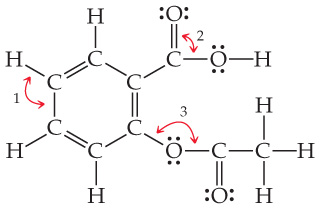# Problem: The compound with the following Lewis structure is acetylsalicylic acid, better known as aspirin: .What are the approximate values of the bond angles labeled 1, 2, and 3?

###### FREE Expert Solution

We’re being asked to determine the approximate values of the bond angles labeled 1, 2, and 3

Recall that electron regions can predict the geometry as well as the bond angles

Electron Regions          Electronic Geometry          Bond Angles

2                                      linear                                    180˚

3                                      trigonal planar                      120˚

4                                      tetrahedral                            109.5˚

5                                      trigonal bipyramidal              90˚, 120˚, and 180˚

6                                      octahedral                            90˚ and 180˚

91% (446 ratings)###### Problem Details

The compound with the following Lewis structure is acetylsalicylic acid, better known as aspirin:.

What are the approximate values of the bond angles labeled 1, 2, and 3?

Frequently Asked Questions

What scientific concept do you need to know in order to solve this problem?

Our tutors have indicated that to solve this problem you will need to apply the Bond Angles concept. You can view video lessons to learn Bond Angles. Or if you need more Bond Angles practice, you can also practice Bond Angles practice problems.

What professor is this problem relevant for?

Based on our data, we think this problem is relevant for Professor Hoeger's class at UCSD.